# Locus of the Sum / Difference of squares of distances from two fixed points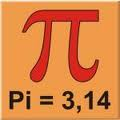Los loci for determining points satisfying a certain geometric condition. Of interest in the resolution of problems in which metric or geometric restrictions.

Some loci are elementary and serve to define geometric shapes known, while others require elaborate processes determining.

So, for example, the locus of points in the plane whose distance from a fixed point is constant is a circle with center point and radius referred to the distance given.

## Relations in the triangle

The direct application of Pythagorean theorem we can obtain some loci high interest in the development of advanced theorems metric geometry.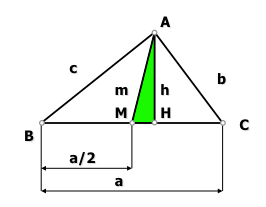The figure has the triangle ABC and have obtained, on the side “to“, the midpoint “M” and walk-up “H” to determine their height “h” from the apex “A“. This allows the determination of three triangles (a right angle) we can relate to each other for two major loci.

The triangles to which we refer are:

• AHB
• AHC
• AHM

As shown in Figure, three triangles share the side “AH” as one of its legs, and the other leg is on the side “to”, base, Triangle; Triangles are longer than the side “AH” is the height of the triangle and thus is perpendicular to said base.

Applying theorem Pythagoras, we obtain the following three ratios: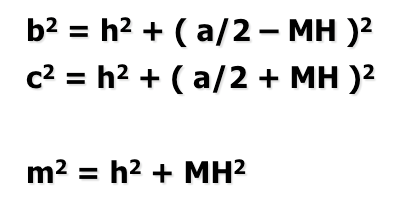adding the first two have the sum of two squareswhereas if we subtract one another have the difference of two squares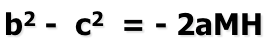## Locus of points whose difference of squares of distances from two fixed points is constant.

Let's see how we can use the above relations to determine the locus of points in the plane that meet the difference of the square of its distance from two fixed points is constant. This theorem we determine may be stated as follows:

The locus of points whose difference of the squares of the distances from two fixed points B and C is a constant quantity k is a line orthogonal to BC whose distance from the midpoint of BC is d = K/2BC.

Suppose that one of the points of the plane that fulfills this condition is the vertex “A” Triangle ABC, and fixed points to which we refer are “B” and “C“.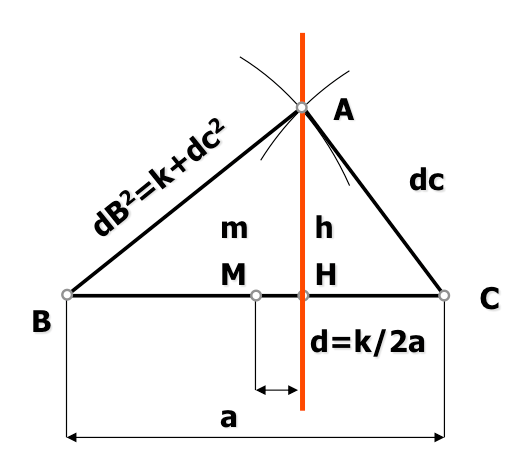From the above relations we can use to express the difference of squares of distances to points “B” and “C“, and impose the condition that this ratio remains constant.
Being fixed the distance between the points “B” and “C“, side value "toconstant. For equality to the equation expressing distance constant "MH"Has to be as well as “tounchanged and “2” is a number that does not change.
We see that the segment "MH" is the projection of the median “m” (straight line connecting a vertex to the midpoint “M” opposite) the segment "BC
This implies that the point “A” can be in any position so that the plane of the median projection on "BC"Remains constant; point "A"Has to move on so The straight "h”, so that the locus that should be searched straight.
This will determine the locus “radical axis” of two circles as seen in the study of orthogonality.

## Locus of points whose sum of squares of distances from two fixed points is constant.

Expression obtained for the sum of squares:it follows that, being “to” constant, so that it is the expression, must be the value “m” the median also a fixed value, thus concluded that the locus must be a circle of radius the value of the median.